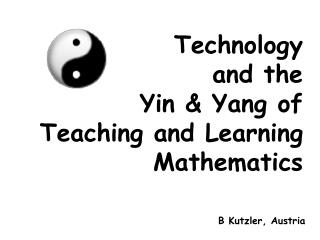DownloadDownload PresentationTechnology and the Yin & Yang of Teaching and Learning Mathematics

# Technology and the Yin & Yang of Teaching and Learning Mathematics

Download Presentation## Technology and the Yin & Yang of Teaching and Learning Mathematics

- - - - - - - - - - - - - - - - - - - - - - - - - - - E N D - - - - - - - - - - - - - - - - - - - - - - - - - - -
##### Presentation Transcript

1. Technology and the Yin & Yang ofTeaching and Learning Mathematics B Kutzler, Austria

2. Humans are ruled by two opposite forces: hold on let go

3. Humans are ruled by two opposite forces: hold on (yin) let go (yang)

4. In our context these show as hold on = connect let go = automate

5. Automate Automate = compensate + amplify = compensate + (solve + explore)

6. automate compensate explore solve

7. Connect Connect= = represent + document + communicate

8. Connect Connect = model  = represent + document + communicate model

9. Connect Connect = model model   = represent + document + communicate  model human

10. Connect Connect = model model human  = represent + document + communicate   model human human

11. connect document communicate represent

12. connect automate document communicate compensate represent explore solve

13. connect automate document communicate compensate represent explore solve

14. Math-Pedagogy-Technology Space technology mathematics pedagogy

15. Math-Pedagogy-Technology Space technology mathematics

16. With a helicopter one can reach places that one cannot reach otherwise.

17. With a helicopter one can reach places that one cannot reach otherwise.B Waits: Tech makes some math possible

18. With a helicopter one can reach places that one cannot reach otherwise.B Waits: Tech makes some math possible Tech makes some math pedagogy possible

19. Not the tool, but the use of the tool is or is not pedagogical. (Vlasta Kokol-Voljc)

20. Teacher training: Step 1: technology axis Step 2: math/technology plane Step 3: math/pedagogy/technology space Introducing teachers to technology needs as much (pedagogical) care as any other teaching

21. (1) Represent

22. (1) Represent Mathematical problem solving = the art of transforming representations (until the solution is visible)

23. (1) Represent Mathematical problem solving = the art of transforming representations (until the solution is visible)such as transforming 5x-6=2x+15into x=7

24. (1) Represent Algebraic/graphic/numeric 1+2  3 3a+4a  7a 24  2 6 24  4.89898

25. (1) Represent Use technology to simulate a mathematician‘s mind New pedagogy (helps more students to understand)

26. (2) Document = share your work with an anonymous person argue (= convince sb with certain level of knowledge) designdescribe

27. (2) Document Describe/understand Newspaper articles Mathematical logic27 is divisible by 3  x(3x=27) every number is greater than its predecessor x(x>x-1) Use wordproblems to practice understanding

28. (2) Document Argue - Examples such asExplain why one uses the first derivative to find the extreme values of a function If a curve is symmetric in both the x- and y-axes, is it then symmetric through the origin? Give a reason or find a counter-example.

29. (2) Document With technology: Result loses importance Choosing keys/commands is mathematical work New pedagogy (shift focus fromexecuting algorithms to documenting mathematical work)

30. (3) Communicate = impart or transmit idea, knowledge, information Documentation = „offline“ communication „Online“ communication

31. (3) Communicate Student  teacher: - front teaching - teacher as advisor and assistant Student  student: - alone (cf traditional exam) - teamwork New pedagogy (enables new teaching/learning styles)

32. (4) Compensate

33. (4) Compensate

34. (4) Compensate

35. (4) Compensate

36. (4) Compensate

37. (4) Compensate